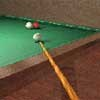#### You may also like### Rain or Shine

Predict future weather using the probability that tomorrow is wet given today is wet and the probability that tomorrow is wet given that today is dry.### Squash

If the score is 8-8 do I have more chance of winning if the winner is the first to reach 9 points or the first to reach 10 points?### Snooker Frames

It is believed that weaker snooker players have a better chance of winning matches over eleven frames (i.e. first to win 6 frames) than they do over fifteen frames. Is this true?

# Snooker

##### Age 16 to 18Challenge Level

In a particular snooker tournament, two players play "frames" against each other and the first to win 8 frames wins the match. This is known as a "best of 15" match. Assume that the probability of player A winning each frame is $p$, regardless of who starts. If A does not win the frame then his opponent does (there are no draws).

Suppose $p = 0.4$, then what is the probability that A wins the match? Now suppose that A is very slightly better than his opponent, say $p = 0.55$, what is the probability now of A winning the match? What is the probability of A winning the match when $p = 0.5$?